Absolute Value
Exponents
Order of Operations
Solve
Distributive Property
Percent
Evaluate
100

Find the absolute value of:

|-10|100

When you see the following expression, what is the big number called and what is the little number on top of it called?

24

100

Simplify the following expression: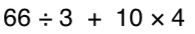100

x - 5x = 64

What is x = -16

100

8(4+2) = ?

What is 48?

100

What is 25% of 80

What is 20?

100

For a = 2, b = 3, c= 4, and d= 12

19 - d

What is 7

200

What is greater:

|-6| or |3|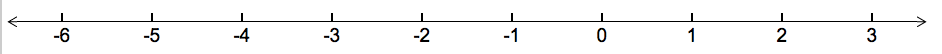The absolute value of a number is the number's distance from zero.

What is l-6 l?

200

How can you rewrite 9in expanded form?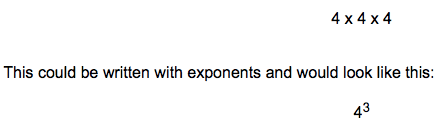What is 9x9?

200

Simplify the following expression: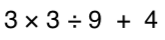200

-0.3m = -12

What is 40?

200

5(x-3)

What is 5x-15?

200

What is 15% of 120

What is 18?

200

For a = 2, b = 3, c= 4, and d= 12

d - c + 6

What is 14?

300

Place the following in order from smallest number, to biggest number:

|-20|, 18, |12|, |-14|, 8

What is 8, l 12 l, l -14 l, 19, l-20 l?

The absolute value of a number is the number's distance from zero.

300

How can you rewrite the following in exponential form?

7 x 7 x 7 x 7 x 7What is 7^4?

300

3 + 42 * (3 + 2)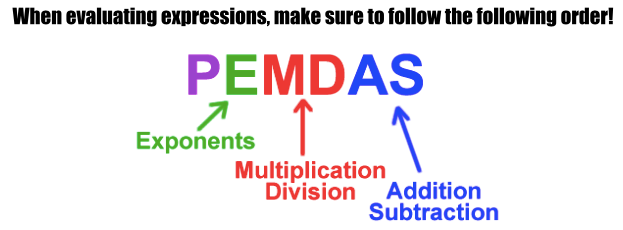What is 95?

300

2.5x = -60

What is -24

300

7+9(2x-3)

What is 18x-20?

300

130% of 42

What is 54.6?

300

For a = 2, b = 3, c= 4, and d= 12

a(d - b)

What is 18?

400

This can be written as:

|-7| + |-2|

The absolute value of a number is the number's distance from zero.

What is 9?

400

What is the value of:

84

400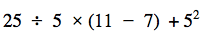What is 45?

400

x - (-3) = 5

What is 2

400

-3(-x-10)

What is 3x+30?

400

8% of 540

What is 43.2?

400

For a = 2, b = 3, c= 4, and d= 12

5a - 2c

What is 4?

500

2 x |-5| + |4| - 14

Remember to use your order of operations.

What is 0?

500

What is the value of:

23 + 32 - 42

500What is 30?

500

x - 0.9x = 7

What is 70?

500

x + 10 -6(x-3x) +4

What is 13x+14?

500

70% of 478

What is 334.6?

500

For a = 2, b = 3, c= 4, and d= 12

d + ac

What is 20

Click to zoom